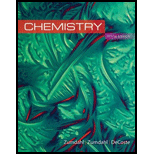# Concentrated hydrogen peroxide solutions are explosively decomposed by traces of transition metal ions (such as Mn or Fe): 2 H 2 O 2 ( a q ) → 2 H 2 O ( l ) + O 2 ( g ) What volume of pure O 2 ( g ), collected at 27°C and 746 torr, would be generated by decomposition of 125 g of a 50.0% by mass hydrogen peroxide solution? Ignore any water vapor that may be present.### Chemistry

10th Edition
Steven S. Zumdahl + 2 others
Publisher: Cengage Learning
ISBN: 9781305957404

#### Solutions

Chapter
Section### Chemistry

10th Edition
Steven S. Zumdahl + 2 others
Publisher: Cengage Learning
ISBN: 9781305957404
Chapter 5, Problem 74E
Textbook Problem
1085 views

## Concentrated hydrogen peroxide solutions are explosively decomposed by traces of transition metal ions (such as Mn or Fe): 2 H 2 O 2 ( a q )   →   2 H 2 O ( l )   +  O 2 ( g ) What volume of pure O2(g), collected at 27°C and 746 torr, would be generated by decomposition of 125 g of a 50.0% by mass hydrogen peroxide solution? Ignore any water vapor that may be present.

Interpretation Introduction

Interpretation:

The volume of O2(g) produced in the given decomposition reaction of 2 moles of H2O2(aq) is to be calculated where the reacted mass of H2O2(aq) is 50% of 125g hydrogen peroxide solution.

Concept introduction:

• According to ideal gas equation for finding volume of a gas,

Volume=Numberofmoles×R×Temperaturepressure

• Mole ratios between the reactants of a reaction are depends upon the coefficients of respective reactant in a balanced chemical equation.
• Number of moles of a substance from its given mass is,

Number of moles=GivenmassMolecularmass

### Explanation of Solution

Explanation

The given reacted mass of H2O2(aq) is 50% of 125g hydrogen peroxide solution. So the mass of H2O2(aq) is 62.5g (half of 125)

Number of moles of a substance from its given mass is,

Number of moles=GivenmassMolecularmass

Therefore, the number of moles of H2O2(aq) reacted is,

Number of molesof H2O2=62.5g*1mole34.02g

Hence, the number of moles of oxygen reacted is 1.8371 mol.

The balance equation of given reaction is 2H2O22H2O+O2, here 1 mole of O2(g) is produced by 2 moles of H2O2(aq) in the given reaction. So 1/2 mole of O2(g) is reacted for 1 mole of H2O2(aq) in the given reaction.

The number of moles of H2O2(aq) is reacted in the given reaction is calculated from the given reacted mass of H2O2(aq) is 1.8371 mol. The molar ratio of O2(g) in the given reaction is 1/2 moles of O2(g) for 1 mole of H2O2(aq).

Therefore, the number of number of moles of O2(g) is needed for given reaction is,

Number of molesofO2 =12×1

### Still sussing out bartleby?

Check out a sample textbook solution.

See a sample solution

#### The Solution to Your Study Problems

Bartleby provides explanations to thousands of textbook problems written by our experts, many with advanced degrees!

Get Started

Find more solutions based on key concepts
In many people any kind of stress can cause overeating and weight gain T F

Nutrition: Concepts and Controversies - Standalone book (MindTap Course List)

What is a joint?

Human Biology (MindTap Course List)

Why are titanium oxide features visible in the spectra of only the coolest stars?

Horizons: Exploring the Universe (MindTap Course List)

The price of gasoline at a particular station is 1.5 euros per liter. An American student can use 33 euros to b...

Physics for Scientists and Engineers, Technology Update (No access codes included)